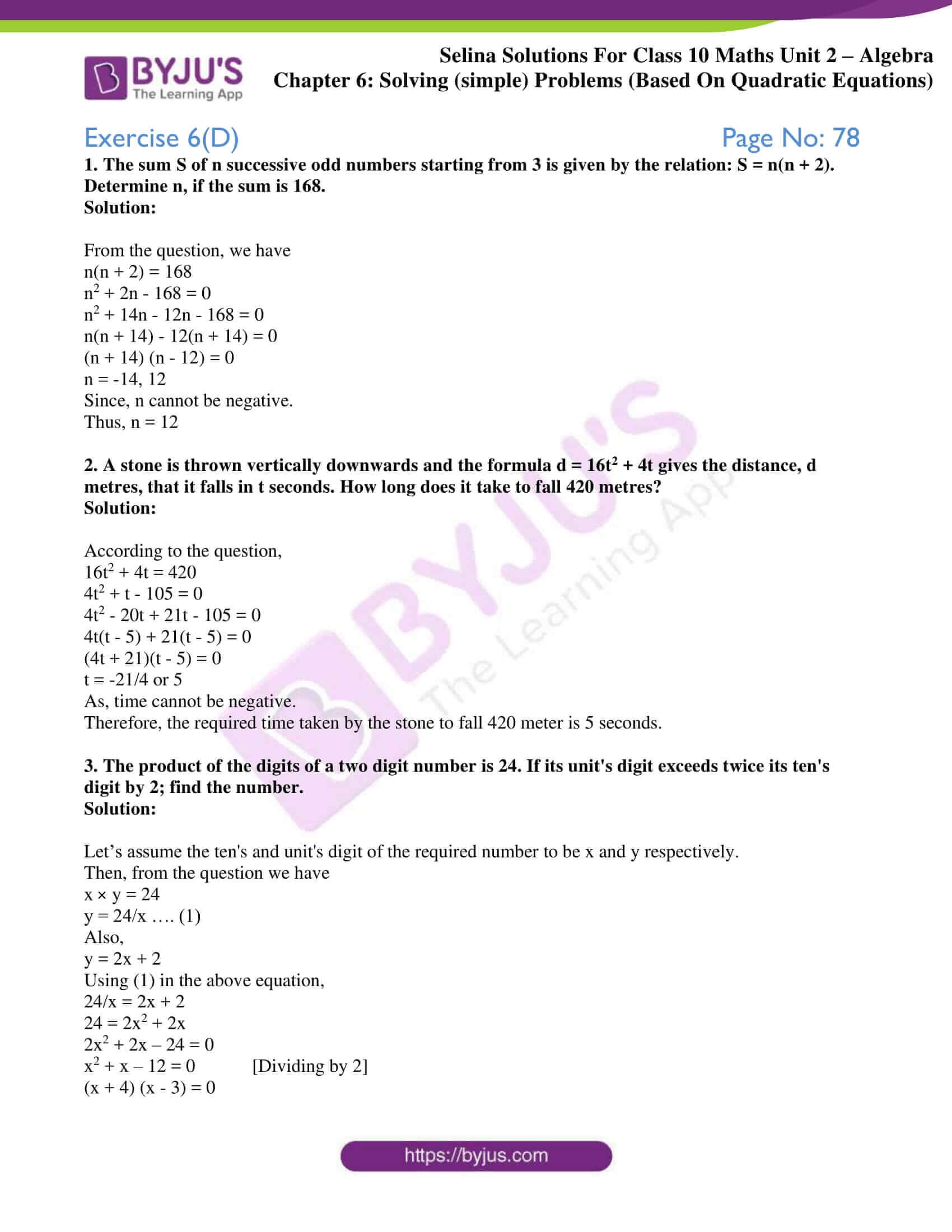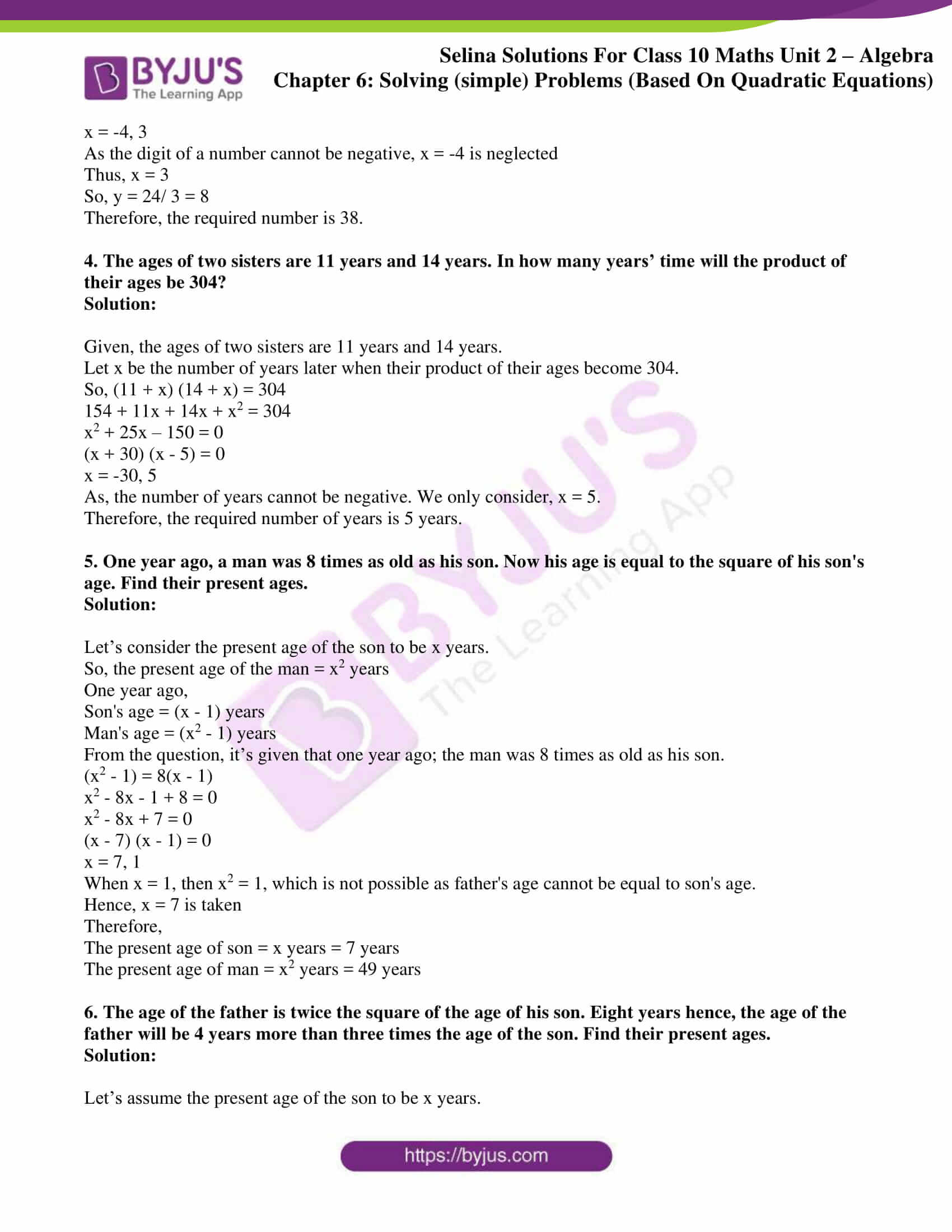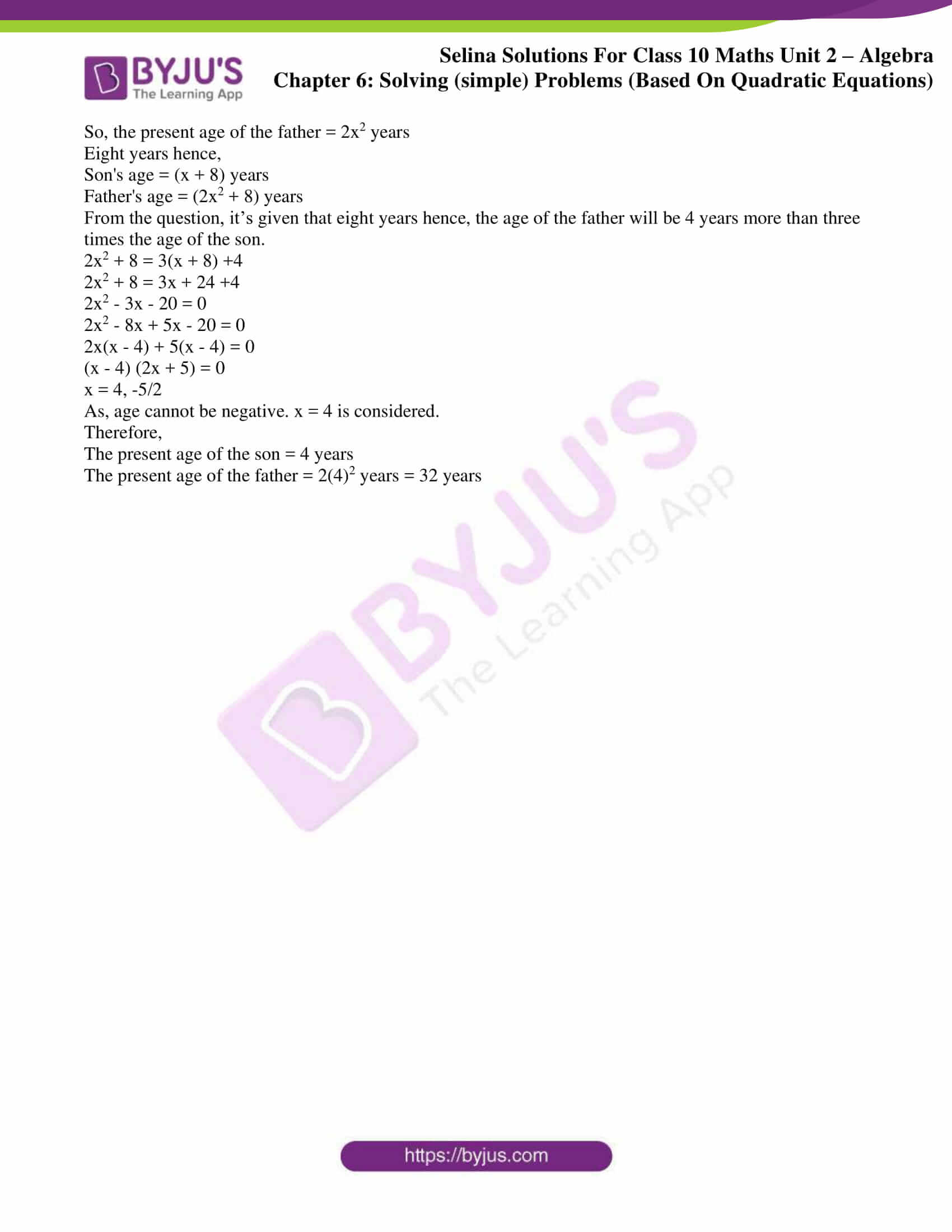# Selina Solutions Concise Maths Class 10 Chapter 6 Solving (simple) Problems (Based On Quadratic Equations) Exercise 6(D)

Quadratic equations have enormous applications in the real world. In this exercise, the students will solve several other miscellaneous problems involving quadratic equations. For all these problems the Selina Solutions for Class 10 Maths contains solutions in a comprehensive manner to meet all the needs of the students. For further references to solutions of this exercise the Concise Selina Solutions for Class 10 Maths Chapter 6 Solving (simple) Problems (Based On Quadratic Equations) Exercise 6(D) PDF is available in the links below.

## Selina Solutions Concise Maths Class 10 Chapter 6 Solving (simple) Problems (Based On Quadratic Equations) Exercise 6(D) Download PDF### Access other exercises of Selina Solutions Concise Maths Class 10 Chapter 6 Solving (simple) Problems (Based On Quadratic Equations)

Exercise 6(A) Solutions

Exercise 6(B) Solutions

Exercise 6(C) Solutions

Exercise 6(E) Solutions

### Access Selina Solutions Concise Maths Class 10 Chapter 6 Solving (simple) Problems (Based On Quadratic Equations) Exercise 6(D)

1. The sum S of n successive odd numbers starting from 3 is given by the relation: S = n(n + 2). Determine n, if the sum is 168.

Solution:

From the question, we have

n(n + 2) = 168

n2 + 2n – 168 = 0

n2 + 14n – 12n – 168 = 0

n(n + 14) – 12(n + 14) = 0

(n + 14) (n – 12) = 0

n = -14, 12

Since, n cannot be negative.

Thus, n = 12

2. A stone is thrown vertically downwards and the formula d = 16t2 + 4t gives the distance, d metres, that it falls in t seconds. How long does it take to fall 420 metres?

Solution:

According to the question,

16t2 + 4t = 420

4t2 + t – 105 = 0

4t2 – 20t + 21t – 105 = 0

4t(t – 5) + 21(t – 5) = 0

(4t + 21)(t – 5) = 0

t = -21/4 or 5

As, time cannot be negative.

Therefore, the required time taken by the stone to fall 420 meter is 5 seconds.

3. The product of the digits of a two digit number is 24. If its unit’s digit exceeds twice its ten’s digit by 2; find the number.

Solution:

Let’s assume the ten’s and unit’s digit of the required number to be x and y respectively.

Then, from the question we have

x × y = 24

y = 24/x …. (1)

Also,

y = 2x + 2

Using (1) in the above equation,

24/x = 2x + 2

24 = 2x2 + 2x

2x2 + 2x – 24 = 0

x2 + x – 12 = 0 [Dividing by 2]

(x + 4) (x – 3) = 0

x = -4, 3

As the digit of a number cannot be negative, x = -4 is neglected

Thus, x = 3

So, y = 24/ 3 = 8

Therefore, the required number is 38.

4. The ages of two sisters are 11 years and 14 years. In how many years’ time will the product of their ages be 304?

Solution:

Given, the ages of two sisters are 11 years and 14 years.

Let x be the number of years later when their product of their ages become 304.

So, (11 + x) (14 + x) = 304

154 + 11x + 14x + x2 = 304

x2 + 25x – 150 = 0

(x + 30) (x – 5) = 0

x = -30, 5

As, the number of years cannot be negative. We only consider, x = 5.

Therefore, the required number of years is 5 years.

5. One year ago, a man was 8 times as old as his son. Now his age is equal to the square of his son’s age. Find their present ages.

Solution:

Let’s consider the present age of the son to be x years.

So, the present age of the man = x2 years

One year ago,

Son’s age = (x – 1) years

Man’s age = (x2 – 1) years

From the question, it’s given that one year ago; the man was 8 times as old as his son.

(x2 – 1) = 8(x – 1)

x2 – 8x – 1 + 8 = 0

x2 – 8x + 7 = 0

(x – 7) (x – 1) = 0

x = 7, 1

When x = 1, then x2 = 1, which is not possible as father’s age cannot be equal to son’s age.

Hence, x = 7 is taken

Therefore,

The present age of son = x years = 7 years

The present age of man = x2 years = 49 years

6. The age of the father is twice the square of the age of his son. Eight years hence, the age of the father will be 4 years more than three times the age of the son. Find their present ages.

Solution:

Let’s assume the present age of the son to be x years.

So, the present age of the father = 2x2 years

Eight years hence,

Son’s age = (x + 8) years

Father’s age = (2x2 + 8) years

From the question, it’s given that eight years hence, the age of the father will be 4 years more than three times the age of the son.

2x2 + 8 = 3(x + 8) +4

2x2 + 8 = 3x + 24 +4

2x2 – 3x – 20 = 0

2x2 – 8x + 5x – 20 = 0

2x(x – 4) + 5(x – 4) = 0

(x – 4) (2x + 5) = 0

x = 4, -5/2

As, age cannot be negative. x = 4 is considered.

Therefore,

The present age of the son = 4 years

The present age of the father = 2(4)2 years = 32 years